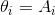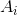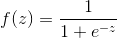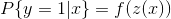Sunday, June 7, 2015

Logistic regression in Excel

Yes, that is weird :) If you need to deal with statistics you have to use a special software like Mathlab or Statistica. But if you are limited in your choice and Excel is the only instrument you have, this manual is for you :)

Linear regression

First of all, Excel already has the "Regression" add-in which allows you to perform a simple lineral regression analysis:

Unfortunately, logistic regression isn't supported by that add-in. But there is always a way to workaround a problem!

Solver

We're starting our journey from an another add-in which name is "Solver". This add-in allows us to solve different minimization/maximization tasks.

Here is an example of a simple maximization problem: we have a furniture factory which produces 2 models of cabinet (A and B). Each model requires a different quantity of resources (wood, time) and generates different income. Also the factory has a limited quantity of available woods and (of course) time. Using simplex-like methods Solver allows us to maximise a target function (the sum of potential income, in current case) by determining the quantity of products for each model that should be produced.

The same idea (solving a maximization task) could be used to compute logistic regression. Let's see how it can be done.

Logistic regression

Imagine that you are an owner of a company and you have a database of clients. Some of them terminated the contract with your company during the last year and you want to predict which clients are thinking about leaving you right now :)

Let's say that you think that decision to stay with your company depends on client's sex and age. Then you can extract the follwing data for the previous year (Trainig Set):

Our next step makes a proposal about how the objective function should look like. In common case it looks like. We assume that there is a linear dependency between decision to leave and sex/age of a client:. In other words, let's just put(theta is a canonical letter for this case, but using it in Excel is a little bit difficult).

The calculation below requires some "initial" values forvalues. For the moment, let's put them eqauls 1 (the values will differ from the ones you see here).
After that we have to calculate the logit function; The main advantage of this function is tending to 0 for x < 0 and tending to 1 for x > 0. So, we can say thatand. Taking into account that y belongs to {0, 1},(Bernoulli distribution).

Hence, our task has been reduced to the selection of the theta () parameters of Z of the objective function Z to maximize P{y|x} probability.

There are two important moments:
Finally, when we foundvalues, we can resote the objective function Z = -25 + 0.586549634 * Age - 1.66748138716445 * Gender.

Let's check the result on our training set:

Where the "Has Terminated" column above is the logit function (ROUND(1/(1+EXP(-T5)),0)).

Now we're sure that our parameters are correct and we can use them to "predict" the future. So, let's do that!

Conclusion

As you can see, the way was a little bit tricky. But anyway, we've managed to pass it! :)